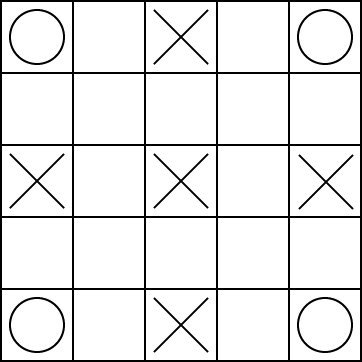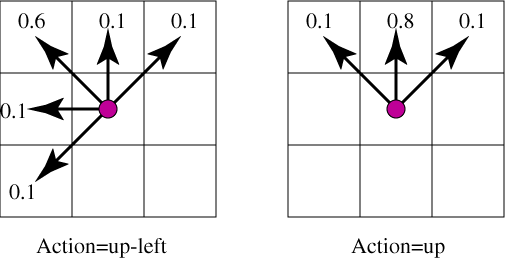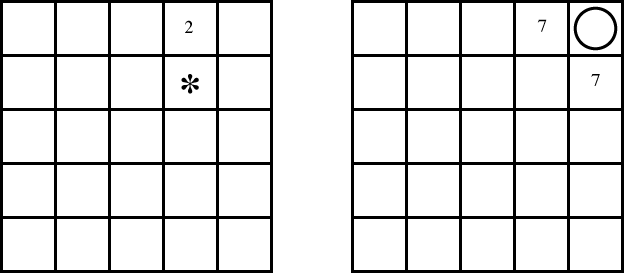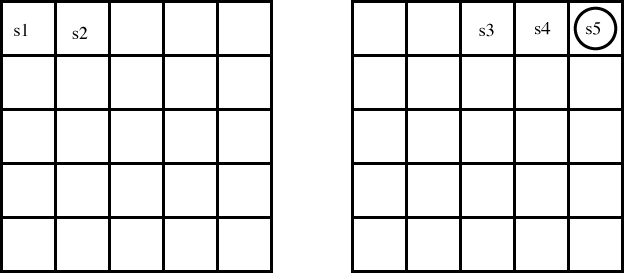CPSC 502 -- Fall 2006
Non-Assignment 5

This is not an assignment in the sense that it will not be marked. I will, however, assume that you have done it when I am setting the midterm. A solution will be posted.

# Question 1

Consider the planning domain in the textbook.
1. Give the feature-based representation of the MW and RHM features.
2. Give the STRIPs representations for the pickup mail, and the deliver mail actions.
3. What are the errors on lecture 11.2, page 4?
4. What are the errors on lecture 11.3, page 5?
5. Suppose the robot cannot carry both coffee and mail at the same time. Give two different ways that the CSP that represents the planning problem can be changed to reflect this. Test it, by giving a problem where the answer is different when the robot has this limitation than when it doesn't.

# Question 2

These questions are available from the textbook available on the course WebCT site.
1. Do exercise 12.2
2. Do exercise 12.4
3. Do exercise 12.5

# Question 3

1. Suppose our Q-learning agent, with fixed alpha (alpha), and discount gamma (gamma), was in state 34 did action 7, received reward 3 and ended up in state 65. What value(s) get updated? Give an expression for the new value. (You need to be as specific as possible)
2. In temporal difference learning (e.q. Q-learning), to get the average of a sequence of k values, we let alpha = 1/k. Explain why it may be advantageous to keep alpha fixed in the context of reinforcement learning.
3. Suppose someone suggested using alphak=10.0/(9.0+k). Explain why it is of this form (e.g., why 9+k on the bottom?) Would you expect it to work well? Explain.
4. Explain what happens in reinforcement learning if the agent always chooses the action that maximizes the Q-value. Suggest two ways that can force the agent to explore.
5. In MDPs and reinforcement learning explain why we often use discounting of future rewards. How would an agent act differently if the discount factor was 0.6 as opposed to 0.9.
6. What is the main difference between asynchronous value iteration and standard value iteration? Why does asynchronous value iteration often work better than standard value iteration?
7. What is the relationship between asynchronous value iteration and Q-learning?

# Question 4

Consider the game domain:The robot can be at one of the 25 locations on the grid. There can be a treasure on one of the circles at the corners. When the robot reaches the corner where the treasure is, it collects a reward of 10, and the treasure disappears. When there is no treasure, at each time step, there is a probability P1=0.2 that a treasure appears, and it appears with equal probability at each corner. The robot knows its position and where the treasure is.

There are monsters at the squares marked with an X. Each monster randomly and independently, at each time, checks if the robot is on their square. If the robot is on the square when the monster checks, it has a reward of -10 (i.e., it loses 10 points). At the centre point the monster checks at each time with probability p2=0.4, at the other 4 squares marked with an X, the monsters check at each time with probability p3=0.2.

The robot has 8 actions corresponding to the 8 neighbouring squares. The diagonal moves are noisy; there is a p4=0.6 probability of going in the direction chosen and an equal chance of going to each of the 4 neighboring squares that are closest to the desired direction. The vertical and horizontal moves are also noisy; there is a probability p5=0.8 chance of going in the requested direction and an equal chance of going to one on the adjacent diagonal squares. For example, the actions up-left and up have the following result:If the action would result in crashing in a wall, the robot has a reward of -2 (i.e., loses 2) and does not move.

There is a discount factor of p6=0.9.

Assume that the rewards are immediate on entering a state (i.e., if the robot enters a state where there is a monster, it gets the (negative) reward on entering the state, and if the robot enters the state where there is a treasure it gets the reward on entering the state, even if the treasure arrives at the same time).

1. Suppose we are using the inefficient state space representation with 125 states.

Suppose we are running value iteration, and have the following values for each state: [The numbers in the square represent the value of that state, and where empty squares have a zero value. It is irrelevant to this question how these values got there]:where the treasure is at the circle. There are also states for the treasures at the other four corners.

Consider the next step of value iteration. For state s13, which is marked by * in the above figure, and the action a2 which is "up", what value is assigned to Q[s13,a2] on the next iteration of value iteration? You need to show all working, but don't need to do any arithmetic (i.e., leave it as an expression). Explain each terms in your expression.

# Question 5

Consider the same domain, but where the agent isn't given a model. Suppose that the agent steps through the state space in the order of steps given in the diagram below, (i.e., going from s1 to s2 to s3 to s4 to s5), each time doing a "right" action.Note that in this figure, the numbers represent the order that the robot visited the states. You can assume that this is the first time the robot has visited any of these states.
1. Suppose a monster did not appear at any time during any of these experiences. What Q-values are updated during Q-learning based on this experience? Explain what values they get assigned. You should assume that alphak=1/k.
2. Suppose that, at some later time, the robot revisits the same states: s1 to s2 to s3 to s4 to s5, and hasn't visited any of these states in between (i.e, this is the second time visiting any of these states). Suppose this time, the monster appears so that the robot gets a penalty. What Q-values have their values changed? What are their new values?

David Poole